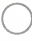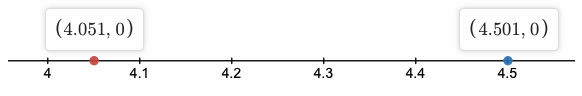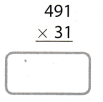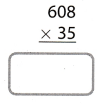Refer to our Texas Go Math Grade 7 Answer Key Pdf to score good marks in the exams. Test yourself by practicing the problems from Texas Go Math Grade 7 Module 14 Answer Key Planning Your Future.

Complete these exercises to review skills you will need for this chapter.

Compare. Write. > or <.

Question 1.
4.0514.501
In the given problem, we have to find out which is greater 4.051 or 4.501
To find which one is greater we will plot both given numbers on the number line and among the given two, the number on the right side of the number line will be greater than the number on the left side of the number line.
So, 4.051 < 4.501Texas Go Math Grade 7 Solutions Module 14 Answer Key Question 2.
73.09073.089
In given problem we have to find out which is greater 73.090 or 73.089
To find which one greater we will plot both given number on the number line and among the given two, the number which on the right side of number line will be greater than the number which on the left side of number line.
So, 73.090 > 73.089Find each product.

Question 3.Given in problem: 716 × 12716 × 12 = 8592Given in problem 491 × 31Question 5.Given in problem 608 × 35Question 6.Given in problem 449 × 52Find each percent.

Question 7.
40% of 30 ________
Given in problem: 40% of 30
We know that value of percentage = $$\frac{\text { Percent }}{100}$$ × Total value
40% of 30 = $$\frac{40}{100}$$ × 30
= 0.4 × 30
= 12

9% of 26 _________
Given in problem: 9% of 26
We know that value of percentage = $$\frac{\text { Percent }}{100}$$ × Total value
9% of 26 = $$\frac{9}{100}$$ × 26
= 0.09 × 26
= 2.34

Question 9.
48% of 130 _________
Given in problem: 48% of 130
We know that value of percentage = $$\frac{\text { Percent }}{100}$$ × Total value
48% of 130 = $$\frac{48}{100}$$ × 130
= 0.48 × 130
= 62.4

Question 10.
66% of 29 _______
Given in problem: 66% of 29
We know that value of percentage = $$\frac{\text { Percent }}{100}$$ × Total value
66% of 29 = $$\frac{66}{100}$$ × 29
= 0.66 × 29
= 19.14

Question 11.
140% of 12 _______
Given in problem: 140% of 29
We know that value of percentage = $$\frac{\text { Percent }}{100}$$ × Total value
140% of 29 = $$\frac{140}{100}$$ × 12
= 1.4 × 12
= 16.8

100% of 584 _______
Given in problem: 100% of 584
We know that value of percentage = $$\frac{\text { Percent }}{100}$$ × Total value
100% of 584 = $$\frac{100}{100}$$ × 584
= 1 × 584
= 584

Visualize Vocabulary

Use the ✓ words to complete the graphic. You will put a different one in each box.Understand Vocabulary

Complete each sentence, using the preview words.

Question 1.
____________________ occur regularly and stay the same.